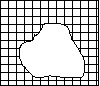# LAF Zone 2: Intuitive Modelling

The information below describes the key characteristics and teaching implications for Zone 2 of the Learning and Assessment Framework for Multiplicative Thinking (LAF), including:

## How to use this resource

There are eight zones in the LAF. If your students are across several zones, you should access information for each of the zones where they are located.

Where there is a reference to:

## Key characteristics

Trusts the count for groups of 2 and 5, that is, can use these numbers as units for counting. For example:

• Tables & Chairs part j
• Butterfly House part d.

Counts large collections efficiently and systematically keeps track of count (for example, may order groups in arrays or as a list) but needs to ‘see’ all groups. For example:

• Tiles, Tiles, Tiles part a
• Butterfly House part e.

May use list and/or doubling as follows:

• 2 butterflies 5 drops
• 4 butterflies 10 drops
• 6 butterflies 15 drops
• 12 butterflies 30 drops

Can share collections into equal groups/parts. For example, Pizza Party parts a and b.

Recognises small numbers as composite units. For example, can count equal group and skip count by twos, threes and fives

Recognises multiplication is relevant, but tends not to be able to follow this through to solution. For example, Packing Pots part c, Speedy Snail part a.

Can list some of the options in simple Cartesian product situations. For example, Canteen Capers part a.

Orders 2 digit numbers. For example, partially correct ordering of times in Swimming Sports part a.

Some evidence of multiplicative thinking as equal groups or shares are seen as entities that can be counted systematically.

## Teaching implications – consolidation and establishment

Consolidate or establish the ideas and strategies introduced or developed in the previous zone.

## Teaching implications – introduction and development

More efficient strategies for counting groups

Introduce strategies based on a change in focus from a count of equal groups (for example, 1 three, 2 threes, 3 threes, 4 threes …) to a consistent number of groups (for example, 3 ones, 3 twos, 3 threes, 3 fours, …) which underpin the more efficient mental strategies listed below and ultimately lead to the factor-factor-product idea.

Array/region-based mental strategies for multiplication facts to 100

For example:

• doubling (for 2s facts)
• doubling and 1 more group (for 3s facts)
• double doubles (for 4s facts)
• relate to tens (for 5s and 9s facts).

For further examples, see There’s More to Counting Than Meets the Eye (pdf - 100.88kb).

Efficient strategies for solving problems where arrays and regions only partially observedFor example:

If paint spill on a tiled floor,

• How many tiles to replace?
• How many altogether?
• How do you know?

Commutativity

Encourage commutativity by exploring the relationship between arrays and regions such as 3 fours and 4 threes.

Informal division strategies

Tasks could include Think of multiplication and halving. For example:

16 divided by 4, think: 4 ‘whats’ are 16? 4; or half of 16 is 8, half of 8 is 4

Extended mental strategies for multiplication

For example:

for 3 twenty fives, think: double 25, 50, and twenty five more, 75

Use place-value based strategies such as 10 groups and 4 more groups for 14 groups.

Simple proportion problems

Involve non-numerical comparisons. For example, If Nick mixed less cordial with more water than he did yesterday, his drink would taste (a) stronger, (b) weaker (c) exactly the same, or (d) not enough information to tell.

How to recognise and describe simple relationships

Encourage recognition of patterns, for example:

• ‘double and add 2’ from models, diagrams and tables
• notice that a diagonal pattern on a 0-99 chart is a count of 11, 1 ten and 1 ones.

Language of fractions

Use practical experience with both continuous and discrete, ‘real-world’ fraction models. For example:

• 3 quarters of the pizza
• half the class.

Distinguish between how many and how much. For example:

in 2 thirds the numeral indicates how many, the name indicates how much

Halving partitioning strategy

Apply thinking involved to help children create their own fraction diagrams through activities such as:

• paper folding (kinder squares and streamers)
• cutting plasticine ‘cakes’ and ‘pizzas’
• sharing collections equally (counters, cards etc).

Focus on making and naming parts in the halving family (for example, 8 parts, eighths) including:

• mixed fractions (for example, “2 and 3 quarters”)
• informal recording (for example, 3 eighths)
• no symbols.

Key fraction generalisations

Use strategies that support the key fraction generalisation that equal parts are necessary and that the number of parts names the part.

## Learning plans

The following learning plans have been developed for this zone, to support targeted teaching after students have been assessed and located on the LAF.